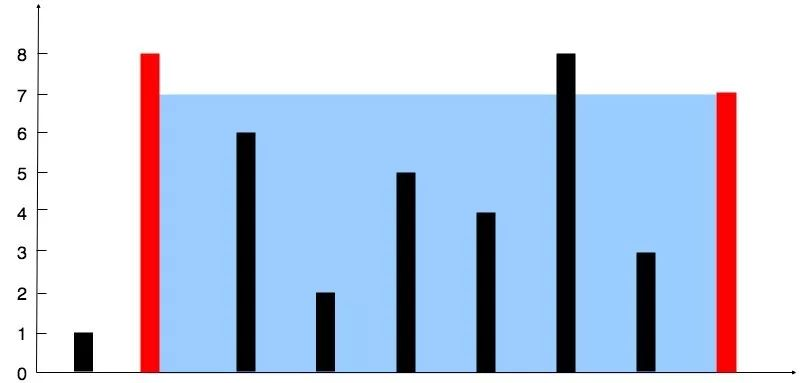# LeetCode.11-装水最多的容器(Container With Most Water)

### 01 看题和准备### 02 第一种解法

public int maxArea(int[] height) {
int result = 0, n = height.length;
for (int i=0; i<n; i++) {
for (int j=i+1; j<n; j++) {
int w = j-i;
int h = Math.min(height[i], height[j]);
result = Math.max(result, w*h);
}
}
return result;
}

### 03 第二种解法

public int maxArea2(int[] height) {
int start = 0, end = height.length-1;
int result = 0;
while (start < end) {
int w = end - start;
int h = Math.min(height[end], height[start]);
result = Math.max(result, w*h);
if (height[start] < height[end]) {
start++;
} else {
end--;
}
}
return result;
}

### 04 第三种解法

public int maxArea3(int[] height) {
int start = 0, end = height.length-1;
int result = 0;
while (start < end) {
int w = end - start;
int h = Math.min(height[end], height[start]);
result = Math.max(result, w*h);
if (height[start] < height[end]) {
start++;
} else if(height[start] > height[end]) {
end--;
} else {
start++;
end--;
}
}
return result;
}

### 05 小结

posted @ 2019-06-11 08:36  程序员小川  阅读(...)  评论(...编辑  收藏